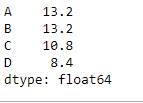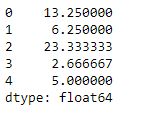# Python | Pandas dataframe.mean()

• Difficulty Level : Medium
• Last Updated : 19 Nov, 2018

Python is a great language for doing data analysis, primarily because of the fantastic ecosystem of data-centric python packages. Pandas is one of those packages and makes importing and analyzing data much easier.

Pandas` dataframe.mean()` function return the mean of the values for the requested axis. If the method is applied on a pandas series object, then the method returns a scalar value which is the mean value of all the observations in the dataframe. If the method is applied on a pandas dataframe object, then the method returns a pandas series object which contains the mean of the values over the specified axis.

Attention geek! Strengthen your foundations with the Python Programming Foundation Course and learn the basics.

To begin with, your interview preparations Enhance your Data Structures concepts with the Python DS Course. And to begin with your Machine Learning Journey, join the Machine Learning - Basic Level Course

Syntax: DataFrame.mean(axis=None, skipna=None, level=None, numeric_only=None, **kwargs)

Parameters :
axis : {index (0), columns (1)}
skipna : Exclude NA/null values when computing the result

level : If the axis is a MultiIndex (hierarchical), count along a particular level, collapsing into a Series

numeric_only : Include only float, int, boolean columns. If None, will attempt to use everything, then use only numeric data. Not implemented for Series.

Returns : mean : Series or DataFrame (if level specified)

Example #1: Use `mean()` function to find the mean of all the observations over the index axis.

 `# importing pandas as pd``import` `pandas as pd`` ` `# Creating the dataframe ``df ``=` `pd.DataFrame({``"A"``:[``12``, ``4``, ``5``, ``44``, ``1``],``                   ``"B"``:[``5``, ``2``, ``54``, ``3``, ``2``], ``                   ``"C"``:[``20``, ``16``, ``7``, ``3``, ``8``],``                   ``"D"``:[``14``, ``3``, ``17``, ``2``, ``6``]})`` ` `# Print the dataframe``df`Let’s use the `dataframe.mean()` function to find the mean over the index axis.

 `# Even if we do not specify axis = 0,``# the method will return the mean over``# the index axis by default``df.mean(axis ``=` `0``)`

Output :Example #2: Use `mean()` function on a dataframe which has `Na` values. Also find the mean over the column axis.

 `# importing pandas as pd``import` `pandas as pd`` ` `# Creating the dataframe ``df ``=` `pd.DataFrame({``"A"``:[``12``, ``4``, ``5``, ``None``, ``1``],``                   ``"B"``:[``7``, ``2``, ``54``, ``3``, ``None``],``                   ``"C"``:[``20``, ``16``, ``11``, ``3``, ``8``],.``                   ``"D"``:[``14``, ``3``, ``None``, ``2``, ``6``]})`` ` `# skip the Na values while finding the mean``df.mean(axis ``=` `1``, skipna ``=` `True``)`

Output :My Personal Notes arrow_drop_up